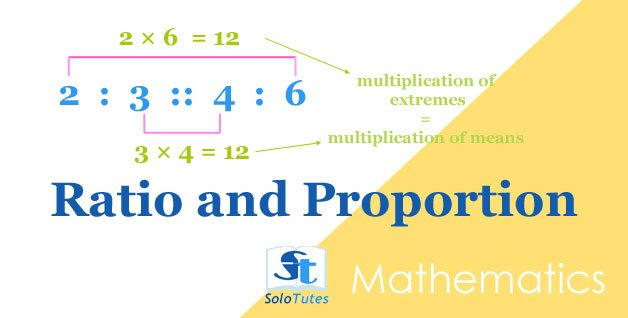SoloTutes

Search By Topics

Followers ×
##### math practice
Lessonsolotutes •
7 Apr 2021## Ratio and Proportion, Basic Tricks and Practice Problems

ratio  can be written in the form of a :b ( where a and b are integers) is the simplified fractional relation ( a/b)  between two quan

Quizsolotutes •
7 Apr 2021

## Ratio

Solve these practice problems on ratio and proportion.

1 of 3 〉 What number has 5 to 1 ratio to the number 10?
• 42
• 55
• 50
• 60
2 of 3 〉 There are 120 students in a picnic tour, if ratio of girls and boys is 5 : 7 then how many boys are in this tour?
• 50
• 70
• 55
• 120

1 more 👇

Quizprakash joshi •
26 Jun 2021## Practice Problems on Average | Math Quiz

To find an average of quantities of the same kind is to add all the items together and then divide the sum by the number of items.

1 of 3 〉 The average weight of A, B and C is  45 kg, that of A and B is 40 kg and B and C is 43 kg. What is the weight of B?
• 17 kg
• 26 kg
• 20 kg
• 31 kg
2 of 3 〉 The average of following 5 numbers is 8, find the missing number in 7, 5, 3,12,?.
• 14
• 13
• 8
• 12

1 more 👇

🗘

### Do you Want to Write Academic Notes Here?

Join SoloTutes.com Telegram Channels@pharmacy_exams@nurseexams@solotutes
##### Visual Stories